previous <<==>> next

 T.I.M. " TIME - IS - MONEY "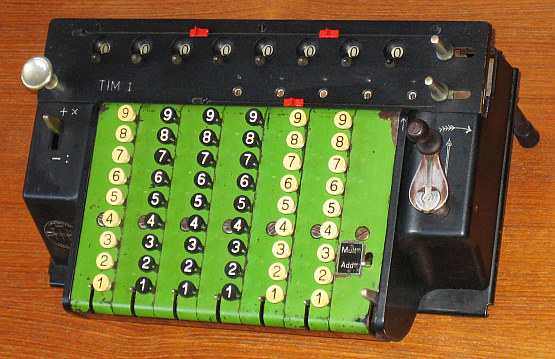Have a look at the Restoration Procedure ... Have a look at the T.I.M. - Model III ...
 ``` TECHNICAL DATA of the Mechanical Calculator » T.I.M. « - Model I ************************************************************************** ( = " TIME - IS - MONEY " ) Serial Number: 30141 Dimensions: (ca.) Width = 12_1/2" / 32 cm Depth = 8_1/4" / 21 cm Height = 5 " / 13 cm Weight: (ca.) 17_3/4 lbs / 8 kg Mechanics: StepDrum / Staffelwalze Functions: Add, Subtract, Multiply, Divide Registers: Input = 6 Decimals (= Keyboard ) Counter = 5 Decimals (NO Carry; Neg.Figures RED) Arithmetic = 8 Decimals Manufacturer: LUDWIG SPITZ & Co. Berlin / Tempelhof Germany 1920 H O W T O U S E the » T.I.M. « ************************************** BEFORE STARTING A TASK: ----------------------- Turn the CRANK to idle POSITION (see picture). CLEAR the whole KEYBOARD by pulling the RIGHT LEVER on front. ( Single COLUMN is cleard with its COLUMN LEVER ) CLEAR the ARITHMETIC & COUNTER UNIT by o LIFTING the CARRIAGE and ... o ... PULL to the RIGHT: UPPER Handle & LOWER Handle LIFT & SHIFT the CARRIAGE to the far left ( = POSITION 1). ADDITION & SUBTRACTION: ----------------------- Example: 123 + 45 - 6 = 162 ADDITION: SET the LEFT LEVER to (+) and the SMALL RIGHT LEVER to (ADD). Enter the first number (123) in the far right columns. Make a (clockwise only!) turn with the crank to transfer the number into arithmetic unit. The counting unit displays the figure 1. Enter the second number (45). Make a turn with the crank to add the number. The arithmetic unit displays the intermediate sum (168) and the counting unit displays the figure 2. SUBTRACTION: SET the LEFT LEVER to (-) Enter the third number (6). Make a turn with the crank. The arithmetic unit displays the result (162) and the counting unit is decreased by 1. REMARK: NEGATIVE RESULTS are displayed in the arithmetic unit as the COMPLEMENT of the next higher 10, 100, 1000, ... Example: -12 = 99...9988 MULTIPLICATION: --------------- Example: 123 x 45 = 5535 CLEAR counter and arithmetic. SET the LEFT LEVER to (+) and the SMALL RIGHT LEVER to (MULT). Enter multiplicand (123) in the far right columns. The multiplicator (45) has two digits, so the arithmetic unit is moved to position 2 by LIFT & SHIFT the CARRIAGE. Repeat making turns with the crank, until the first figure of the multiplicator (4) will appear in the 2nd position of the counter unit. Move the arithmetic unit by LIFT & SHIFT to position 1. Repeat making turns with the crank, until the second figure of the multiplicator (5) appears in the 1st position of the counter unit. The multiplication is done: The multiplicand (123) stays in keyboard, the multiplicator (45) in the counter unit and the result (5535) is in the arithmetic unit. DIVISION: --------- Example: 22 : 7 = 3.1428 Remainder 4 Division requires 3 steps: (A) To Set the Dividend into Arithmetic Unit: ----------------------------------------- SET the LEFT LEVER to (-/:) and the SMALL RIGHT LEVER to (MULT). For the maximum number of decimals, LIFT & SHIFT the arithmetic to the far right position and ENTER the DIVIDENT (22) in columns 6 & 5 of the ARITHMETIC with the affiliated conic knobs. (B) To Set the Divisor in the Keyboard: ----------------------------------- CLEAR COUNTER & INPUT unit. Enter the divisor (7) in the far right column. (C) To Divide: ---------- Make turns with the crank until the arithmetic shows an "underflow". SET the LEFT LEVER to (+), make a turn with the crank. SET the LEFT LEVER back to (-). LIFT & SHIFT the carriage to the next left position. Repeat this procedure until required number of decimals ... The result (3.1428) is in the counter unit ( in RED ), and the remainder (4) is in the arithmetic unit. The divisor (7) stays in the input unit, therefore an additional decimal can be estimated ... ( 5, cause 4 x 7 = 35). Have a look at "Calculating Trickies" ... R E M A R K S : =========================================================================== The COUNTER-FIGURES ( WHITE/RED ) follows the "FELIKS-TYPE"-Scheme ... ```
 This model is nicknamed "Travel T.I.M." There are bigger "TIMs" with more digits available; also with " S h i f t - I n p u t " instead of a Keyboard see  T.I.M. III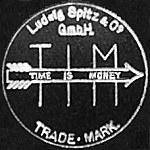```T R A V E L I N G : Reduce HEIGHT of the »T.I.M.« by removing the CRANK --------------------------------------------------------------------------- (1st) Lift SPRING and PULL CRANK HANDLE radially to the rear ... (2nd) ... STORE crank handle in space on LEFT SIDE under the CARRIAGE !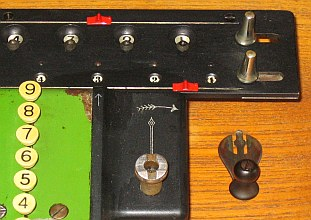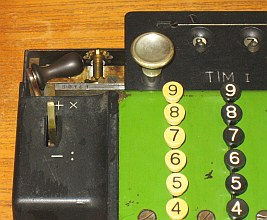M I S S I N G R U B B E R F E E T I N S T A L L E D ... --------------------------------------------------------------------------- (1st) "SHOES" ON THE LEGS, if »T.I.M.« is switched IN UPRIGHT POSITION (2nd) Rubber RINGS ( from GAS HOSE ), mounteded ON THE LEGS "SHOLDERS" (3rd) Two Rubber BLOCKS as FEET, mounted ON BASE FRONT ...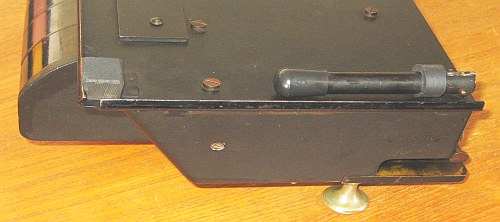Have a look at the Restoration Procedure ... impressum: *************************************************************************** © C.HAMANN http://public.BHT-Berlin.de/hamann 12/13/12 ```